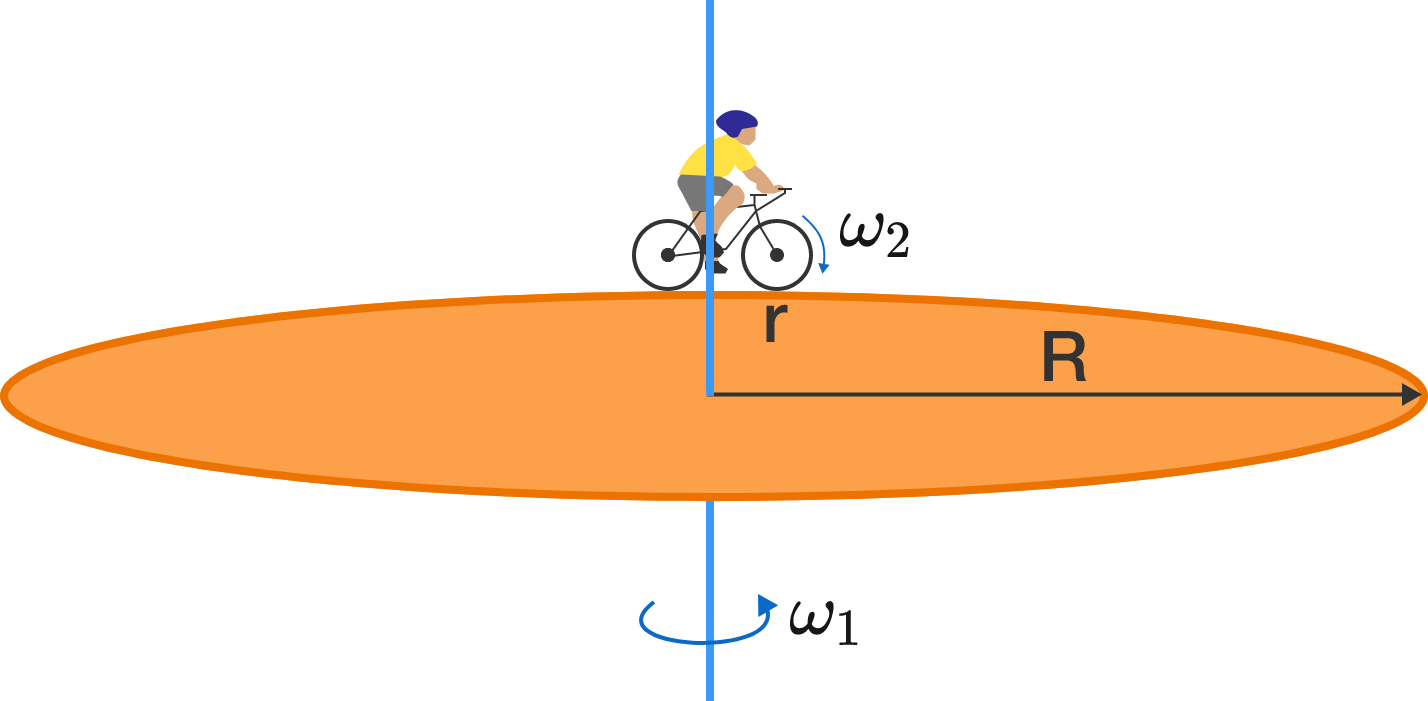# A bicycle on a rotating diskA cyclist is riding a bicycle of wheel radius $r$ along the edge of a rotating disk of radius $R\, (>r)$ in such a way that he appears to be stationary to a person standing on the ground.

If the tires of the bicycle don't slip on the disk (that is, the contact points of the wheel and the disk have equal velocities), then which will have a greater rotating speed?

Clarification: The "rotating speed" is the angular speed (or the magnitude of the angular velocity) given by the rate of change of $\theta$ with respect to the axis about which it is rotating:

$\omega = \dfrac{d\theta}{dt}$

×